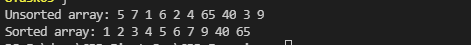Write a program that should have a user-defined function names bubbleSort( ), which should have one parameter of pointer to an integer array. The function should sort the values of that array in ascending order (smaller to larger). Then, in the main( ) function, create an integer array initialized with some values within the code and print the array values along with a message saying that is the original/unsorted array values. After that, pass that array to the function bubbleSort( ) that should sort its values. Finally, print the array values along with a message saying that these are the sorted values.

#include <iostream>
using namespace std;

void bubblesort(int *arr)
{
int temp;
cout << "Unsorted array: ";
for (int i = 0; i < 10; i++)
{
cout << arr[i] << " ";
}
cout << endl;
for (int i = 0; i < 10; i++)
{
for (int j = i+1; j < 10; j++)
{
if (arr[i] > arr[j])
{
temp = arr[j]; // 2 5 6 1
arr[j] = arr[i];
arr[i] = temp;
}
}
}
cout << "Sorted array: ";
for (int i = 0; i < 10; i++)
{
cout << arr[i] << " ";
}

}
int main()
{
int arr[] = {5, 7, 1, 6, 2, 4, 65, 40, 3, 9};

bubblesort(arr);
return 0;
}cpp programming exercise with solution